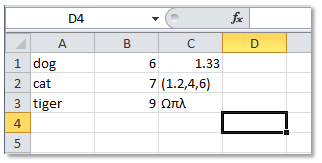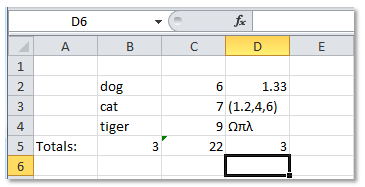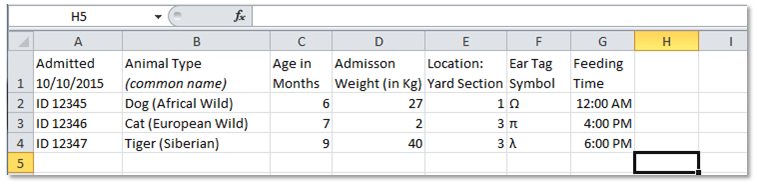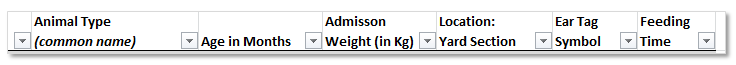What is a Data Structure?  Part 1
Using Tables in Excel 2010Whether you are just going to look at your data and draw conclusions visually, or you are going to write a computer program to manipulate the data, a data structure can help. A data structure is designed to organize data to suit a specific purpose. Typically data to be analysed with software like Excel is stored in a file format that is readable by Excel, and the data are organized in columns to fit into the columns of the spreadsheet. In what follows we will make a distinction between a “spreadsheet” and a “table” because a table  in Excel has special properties.

When analyzing data, it is useful to decide on the data that needs to be processed, and then organize that data into a data structure. A well designed data structure can make the operations that need to be done on the data very efficient.

In Excel, the structure is a grid with columns and rows, without many checks on what goes into the cells. An empty spreadsheet is just a bunch of cells in a grid.We can put just about anything into any cell and Excel won’t complain about it.In order to do analysis on data, we need to have the same type of data in either the rows or columns. The data that we want to do operations on should be grouped together so we can perform meaningful mathematical operations. Many operations will be done on entire columns of data.

Here we have assumed that the data in each row is related and just rearranged the data in the columns:Also, let’s move the data over a cell so that we have some maneuvering room:One thing we can do is get some totals, for example we can count the cells:In cells B5, C5 and D5 we have counted the number of cells in those columns. We know that we have 3 items in column B, 3 in C and 3 in D. However, we could change the total in column C to be a sum, i.e., we are going to add the numbers in that column:There is a limit to the number of operations a person can do based on the data types in a column. For example in D2 we have the number 1.33 while in D3 we have a structure containing 3 numbers and D4 has text of some sort – it doesn’t make sense to add them. In this case we can count the number of cells that have stuff in them but that is about all.

In column C we just have numbers so we can add them, find the average, find minimum and maximum values … we can do a variety of different types of calculations:At this point we would need more information about the data before we can do any meaningful analysis.

Suppose we have checked other sources about the data and we now have the following. (Notice that we have added column names in order to make the data more meaningful.)This is just a simple spread-sheet but Excel does have a data structure called a table which allows us to do additional things with a data set easily. So let’s turn this small example into a table structure and explore the offerings.Note the downward arrows on the right side of each column header cell.This is a drop-down list which allows for both sorting and filtering options. Sorting on a column results in all rows being sorted appropriately. This simplifies sorting compared to when the data isn’t in a table format.

When we look at the drop-down for column B, “Animal Type”:We can see that Excel recognizes that the data in the column is text, and offers to build Text Filter rules.

When we choose the drop-down arrow for Weight:Excel recognizes the data in the column as numbers and offers Number Filters.

Here is the table after we have sorted the weight from smallest to largest:## Summary:

Arranging data in meaningful groups, checking the data, annotating the data and working with table structures allows for quick and simple data exploration.

Why do we care about tables in Excel? They are a nice example of a simple data structure that enables us to do some operations on data easily.

In the world of analytics:

• Users of the R language place tables into a “data frame” structure
• Users of the Python Pandas module use “pandas.DataFrame’s”.
• Both of these structures hold the contents of tables from Excel or databases.

Much of business data comes in tables and once we understand the structure and its limitations we can focus on the analysis in any of a number of tools to achieve the desired results.